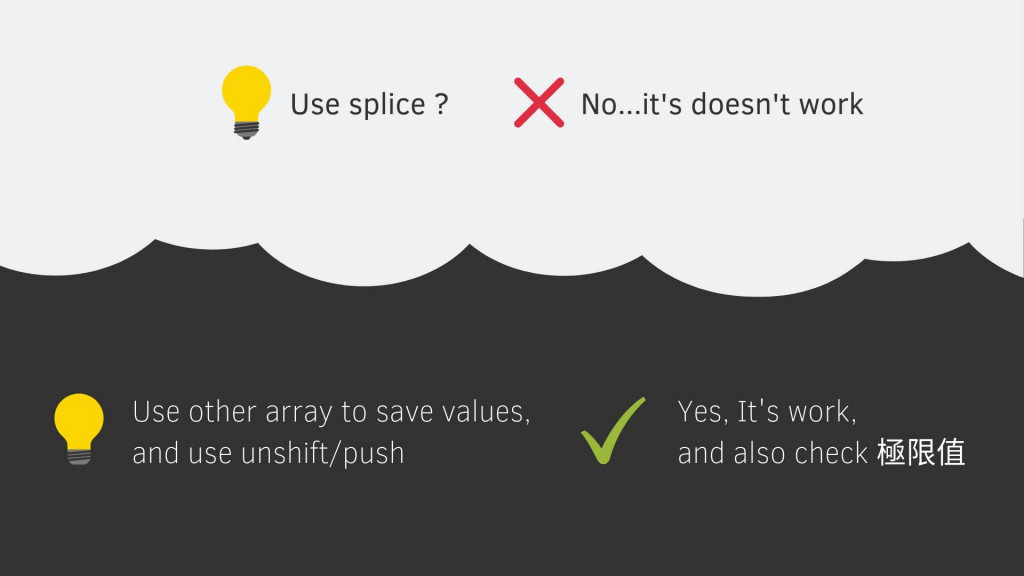#DAY 6
3
Software Development

## 905. Sort Array By Parity

``````/*
Given an array A of non-negative integers,
return an array consisting of all the even elements of A,
followed by all the odd elements of A.

You may return any answer array that satisfies this condition.

input: 給一個沒有負數的數字陣列
output: 回傳前面是偶數後面是奇數的陣列

Example 1:

Input: [3,1,2,4]
Output: [2,4,3,1]
The outputs [4,2,3,1], [2,4,1,3], and [4,2,1,3] would also be accepted.

Note:

1 <= A.length <= 5000
0 <= A[i] <= 5000
*/

/**
* @param {number[]} A
* @return {number[]}
*/
var sortArrayByParity = function(A) {

};
``````

``````Output: [2,4,3,1]  → 先偶數 (even) 再奇數 (odd)
``````

### Think

#### 極限值/特殊狀況

• 可能會有一樣的值 [1, 2, 1]
• 如果只有一個值 
• 值不是數字 ( 但題目已經說一定是數字所以這種狀況就不用再判斷了 )

#### 哪種資料結構解

• 這題很容易想，題目有 Array 又跟排序有關，那當然就是 Array 了

#### 大概會怎麼解• Array 遍歷ㄧ次，發現偶數就從前面放，奇數就從後面放
``````// 大概是這種概念
[3, 1, 2, 4]

[ ] ← 3
 ← 1
2 → [3, 1]
4 → [2, 3, 1]

[4, 2, 3, 1]
``````

#### 用程式實踐

``````var sortArrayByParity = function(A) {
// 先處理極限值
if(A.length < 2){
return A
};
// 把想法變成程式碼實踐
let temp = []
A.forEach( item => {
item%2==0 ? temp.unshift(item) : temp.push(item)
})
return temp
};
``````

Array 遍歷ㄧ次，所以時間複雜度是 Big O(n)

## 561. Array Partition I

``````/*
Given an array of 2n integers, your task is to group these integers into n pairs of integer, say (a1, b1), (a2, b2), ..., (an, bn) which makes sum of min(ai, bi) for all i from 1 to n as large as possible.

input: 給一個偶數的陣列
output: 回傳 min(a1, b1) + ... min(ai, bi) 的最大值

Example 1:
Input: [1,4,3,2]

Output: 4
Explanation: n is 2, and the maximum sum of pairs is 4 = min(1, 2) + min(3, 4).
Note:
n is a positive integer, which is in the range of [1, 10000].
All the integers in the array will be in the range of [-10000, 10000].
*/

/**
* @param {number[]} nums
* @return {number}
*/
var arrayPairSum = function(nums) {

};
``````

### Think

• 有排序嗎？看起來是沒有

#### 哪種資料結構解

• 一樣是 Array，然後會用到 Math.min()

#### 大概會怎麼解

• 我知道加總起來要得到最大值，就是小的跟小的在一起，大的跟大的再一起。所以首先要先排序 (很多題目都要先排序再做)，然後依序加起來。

#### 用程式實踐

``````var arrayPairSum = function(nums) {
const len = nums.length;
let result = 0;
nums = nums.sort((a,b) => a-b);
for(let i= 0; i < len; i += 2){
result += Math.min(nums[i], nums[i+1])
}

return result;
};
``````

### 2 則留言

0

Array Partition I 那題時間複雜度不是 O(n) 喔，因為有用到排序了，所以應該是 O(nlogn)

huli iT邦新手 5 級 ‧ 2019-09-08 00:19:48 檢舉

hannahpun iT邦新手 5 級 ‧ 2019-09-08 00:53:07 檢舉

huli iT邦新手 5 級 ‧ 2019-09-08 01:11:42 檢舉

hannahpun iT邦新手 5 級 ‧ 2019-09-08 01:31:38 檢舉

0

hannahpun iT邦新手 5 級 ‧ 2019-09-10 11:35:01 檢舉